# Circular Arcs, Circles & Angles: Help and Review Chapter Exam

Exam Instructions:

Choose your answers to the questions and click 'Next' to see the next set of questions. You can skip questions if you would like and come back to them later with the yellow "Go To First Skipped Question" button. When you have completed the practice exam, a green submit button will appear. Click it to see your results. Good luck!

### Page 1

#### Question 1 1. In the picture below, if arc BD is 168 degrees and arc BC is 70 degrees, what is the measure of angle BAD?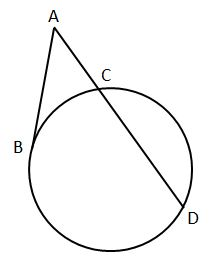#### Question 2 2. In the picture below, if AC is 14 and AB is 10, what is the length of AD?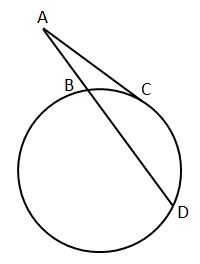#### Question 3 3. In the picture below, if AB is 12, AC is 24 and AD is 10, what is the length of AE?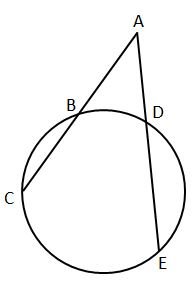#### Question 4 4. In the picture below, if arc CE is 172 degrees and arc BD is 80 degrees, what is the measure of angle CAE?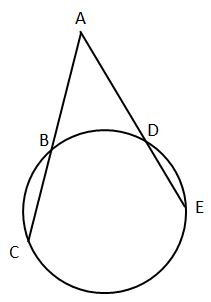#### Question 5 5. In the picture below, if major arc BC is 258 degrees and minor arc BC is 102 degrees, what is the measure of angle BAC?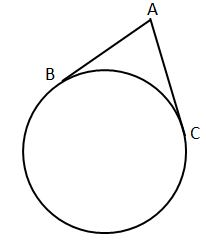### Page 2

#### Question 8 8. If arc BC is 84 degrees, what is the measure of angle ACB?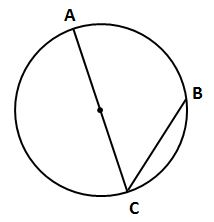#### Question 9 9. In the picture below, if arc AB is 82 degrees, what is the measure of angle BAC?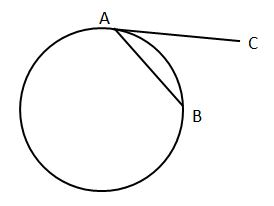### Page 3

#### Question 12 12. If the area of the square is 64, what is the area of the circle, rounded to the nearest whole number?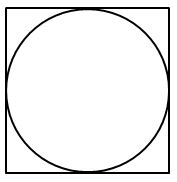#### Question 15 15. If arc AB is 72 degrees, what is the measure of angle ACB?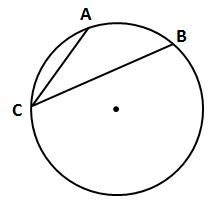### Page 4

#### Question 17 17. What is the area of the shaded area, rounded to the nearest whole number?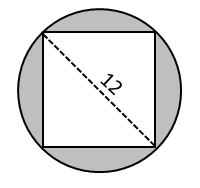#### Question 19 19. Which line is a tangent to the pictured circle?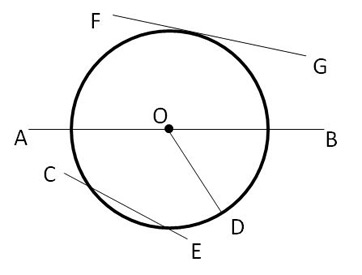### Page 5

#### Question 21 21. If the measure of central angle AOB is 62 degrees, what is the measure of inscribed angle ACB?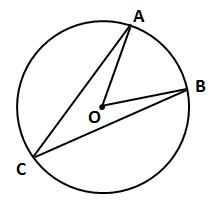#### Question 22 22. What is the area of the shaded area, rounded to the nearest whole number?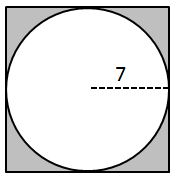#### Question 23 23. In the picture below, if arc AC is 146 degrees and arc BD is 32 degrees, what is the measure of angle AEC?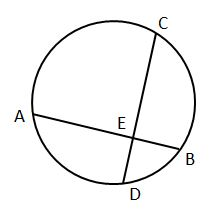#### Question 24 24. In the picture below, if AE is 8, CE is 12 and ED is 5, what is the length of EB?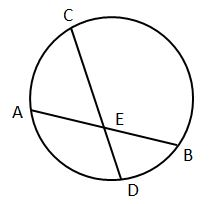#### Question 25 25. In the picture below, if AB is 7, BC is 19 and AD is 8, what is the length of DE?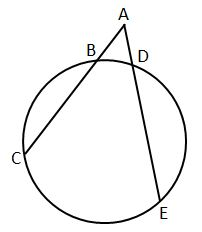### Page 6

#### Question 27 27. If arc AC is 106 degrees and arc BC is 82 degrees, what is the measure of angle ACB?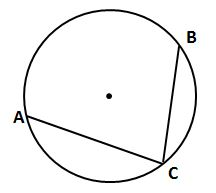#### Circular Arcs, Circles & Angles: Help and Review Chapter Exam Instructions

Choose your answers to the questions and click 'Next' to see the next set of questions. You can skip questions if you would like and come back to them later with the yellow "Go To First Skipped Question" button. When you have completed the practice exam, a green submit button will appear. Click it to see your results. Good luck!

Support